# minimap

A beautiful tile grid map is only a function call away! Tile grid maps are a great way to display geographic data when you want to represent regions with equal visual space. No shapefiles required, just supply a vector of postal abbreviations and a corresponding vector of colors. Built with base graphics.

If you're unfamilar with tile grid maps, check out the examples below or see the following news articles:

## Install

``````library(devtools)
install_github("seankross/minimap")``````

## Demos

``````# La Patria Es Primero

library(minimap)
minimexico(mexico_abb, colorRampPalette(c("#006847", "white", "#CE1126"))(32),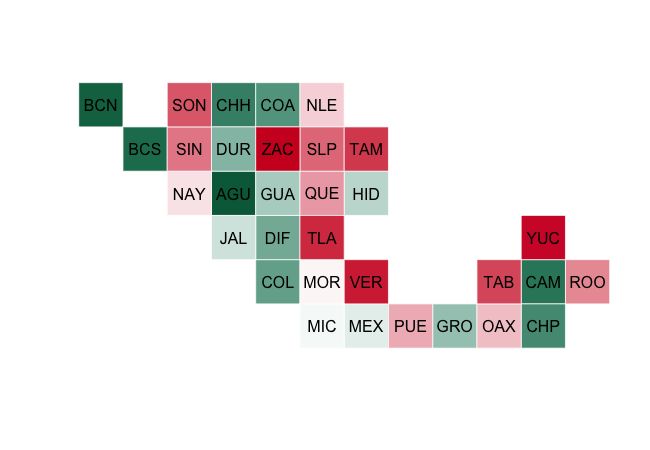Mexico

``````# Legal Status of Same Sex Marriage in the United States (2008)

library(minimap)

determine_color <- function(status){
if(status == "bbs")
"#FFE597"
else if(status == "nl")
"#F1F1F0"
else if (status == "dis")
"#D0C7B9"
else if(status == "bbca")
"#FDC471"
else
"#817972"
}

ssm\$color <- as.character(sapply(ssm\$Status, determine_color))
ssm_2008 <- ssm[ssm\$Year == 2008,]
miniusa(ssm_2008\$State, state_colors = ssm_2008\$color, state_names = TRUE,
state_name_colors = rep("white", 51))
title(main = "Legal Status of Same Sex Marriage in 2008", line = -1)``````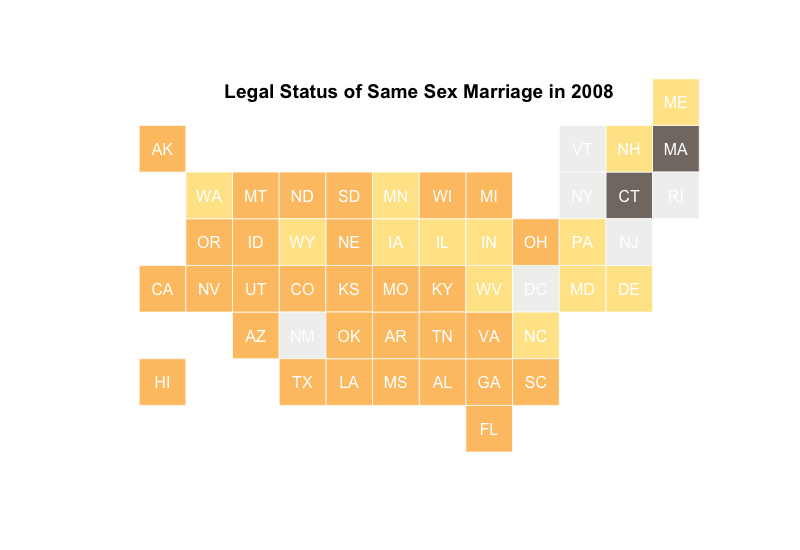USA

``````# Legal Status of Gay Marriage Over Time

library(minimap)

old_mai <- par()\$mai
par(mai = c(0, 0, .75, .5), mfrow = c(2, 4))

for(i in 2008:2015){
one_year <- ssm[ssm\$Year == i,]
miniusa(one_year\$State, state_colors = one_year\$color, state_names = FALSE)
title(main = i, line = -2)
}

mtext("Legal Status of Gay Marriage Over Time", outer = TRUE, side = 3, line = -2)

par(mai = old_mai, mfrow = c(1, 1))``````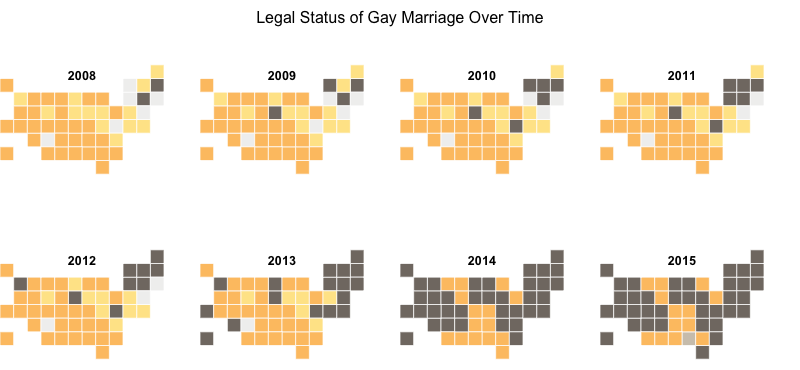USA

``````# Forty Years of Canadian Milk Production

library(minimap)
library(dplyr)
library(RColorBrewer)
library(animation)

bin <- function(x){
qs <- as.numeric(quantile(x, seq(0, 1, .1)))
sapply(x, function(y){
which(abs(qs-y)==min(abs(qs-y)))
})
}

milk_year <- milk %>%
group_by(Region, Year) %>%
summarise(Total_kL = sum(Kiloliters))

max_milk <- milk_year %>%
group_by(Region) %>%
summarise(Max = max(Total_kL))

milk_year <- milk_year %>%
left_join(max_milk) %>%
group_by(Region) %>%
mutate(Bin = bin(Total_kL)) %>%
select(Region, Year, Bin)

missing_milk <- data.frame(Region = rep(missing_pt, each = 40),
Year = rep(1976:2015, 3),
Bin = rep(0, 120), stringsAsFactors = FALSE)
milk_year <- rbind(milk_year, missing_milk)

milk_year\$color <- sapply(milk_year\$Bin, function(x){
if(x == 0){
"grey80"
} else {
brewer.pal(11, "PuOr")[x]
}
})

ani.options(interval = 0.4, ani.width = 600, ani.height = 450)

saveGIF(
for(i in 1976:2015){
milkgif <- milk_year[milk_year\$Year == i,]
minicanada(milkgif\$Region, pt_colors = milkgif\$color, pt_name_cex = 1.5)
title(main = paste("Canadian Milk Production in", i), line = -1)
}
)``````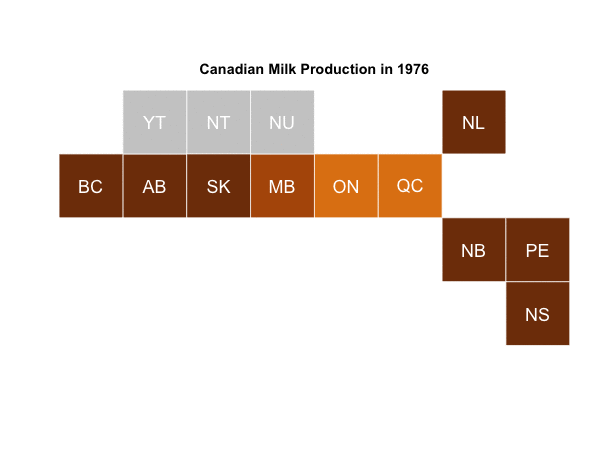``````# Legal Status of Gay Marriage Over Time (gif)

library(minimap)
library(animation)

determine_color <- function(status){
if(status == "bbs")
"#FFE597"
else if(status == "nl")
"#F1F1F0"
else if (status == "dis")
"#D0C7B9"
else if(status == "bbca")
"#FDC471"
else
"#817972"
}

ssm\$color <- as.character(sapply(ssm\$Status, determine_color))

ani.options(interval = 0.5, ani.width = 600, ani.height = 450)

saveGIF(
for(i in 1992:2015){
one_year <- ssm[ssm\$Year == i,]
miniusa(one_year\$State, state_colors = one_year\$color)
title(main = paste("Legal Status of Same Sex Marriage in", i), line = -1)
}
)``````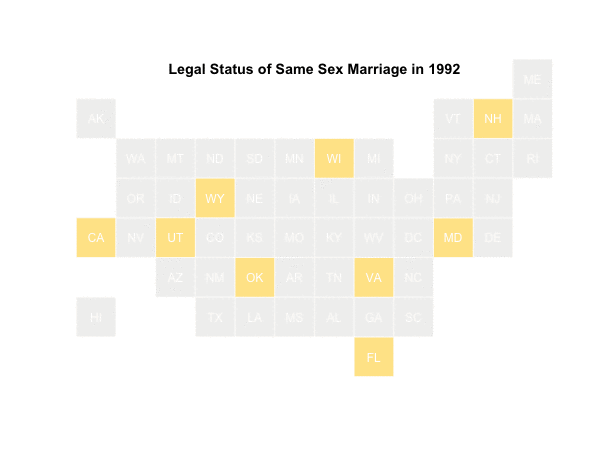USA